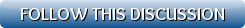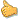[Show all top banners]
 Back to: Kurakani General Discussion Refresh page to view new repliessequence and series
 [VIEWED 3376 TIMES] SAVE! for ease of future access.
anugamanPosted on 10-09-09 4:18 AM     Reply [Subscribe]
Login in to Rate this Post:     0?Given a series a1, a2.......an. If a1 =-4, a2 =5 and an = a(n-2)-a(n-1), then find the sum of first 100 numbers in the series?
[NOTE: 1, 2, (n- 2), (n-1) and n in the above question are subs].

anugamanPosted on 10-09-09 3:12 PM     Reply [Subscribe]
Login in to Rate this Post:     0?anybody?

NightElfPosted on 10-09-09 3:59 PM     Reply [Subscribe]
Login in to Rate this Post:     0?For arithmetic sequence, we have two basic formulas

S = ( n / 2 ) ( A + T )

wherein

S = sum of n terms

n = number of terms

A = first term

T = last term

If the last term is not given instead only the first term is given we use the formula that visibleman_7887 has mentioned

S = ( n / 2 ) [ 2A + ( n - 1 ) * d ]

wherein

S = Sum of n terms

n = number of terms

A = first term

d = common difference

Source:

anugamanPosted on 10-09-09 9:57 PM     Reply [Subscribe]
Login in to Rate this Post:     0?i dunt know how to start. formula only doesnt help.anybody

cowboyPosted on 10-09-09 10:19 PM     Reply [Subscribe]
Login in to Rate this Post:     0?To give u a hint,  the first 100th sum consists of only two terms N2 and N99 since all the other terms cancel out. There is a very easy method to calculate it. The only terms involved in the final calculation depend upon N1 and N2 only. It's not even necessary to calculate N99.

If it's not a physics problem but a numerical analysis problem then you can solve it by writing a simple using either iteration or recursion. It would be similar to solving a Fibonacci Series.

-Cowboy

Last edited: 09-Oct-09 10:25 PM

cowboyPosted on 10-10-09 7:48 PM     Reply [Subscribe]
Login in to Rate this Post:     0?Another hint. The final solution is: 2*N2 - N1. I would like you to figure out how it's derived tho.

Regular summation formulas can't be used since the sequence is not monotonous.